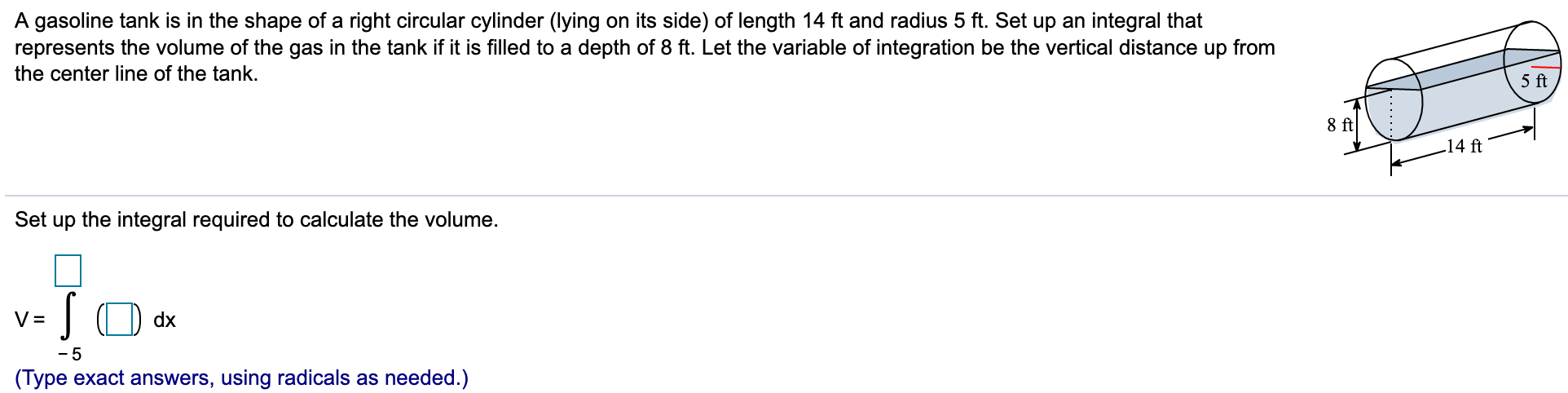# A gasoline tank is in the shape of a right circular cylinder (lying on its side) of length 14 ft and radius 5 ft. Set up an integral thatrepresents the volume of the gas in the tank if it is filled to a depth of 8 ft. Let the variable of integration be the vertical distance up fromthe center line of the tank.5 ft8 ft14 ftSet up the integral required to calculate the volumedx-5(Type exact answers, using radicals as needed.)

Question
54 views

I need help for this.help_outlineImage TranscriptioncloseA gasoline tank is in the shape of a right circular cylinder (lying on its side) of length 14 ft and radius 5 ft. Set up an integral that represents the volume of the gas in the tank if it is filled to a depth of 8 ft. Let the variable of integration be the vertical distance up from the center line of the tank. 5 ft 8 ft 14 ft Set up the integral required to calculate the volume dx -5 (Type exact answers, using radicals as needed.) fullscreen
check_circle

Step 1

Let x be the vertical distance up from the center line of tank ,

So,

Step 2

So, width of a rectangul...

### Want to see the full answer?

See Solution

#### Want to see this answer and more?

Solutions are written by subject experts who are available 24/7. Questions are typically answered within 1 hour.*

See Solution
*Response times may vary by subject and question.
Tagged in

### Calculus